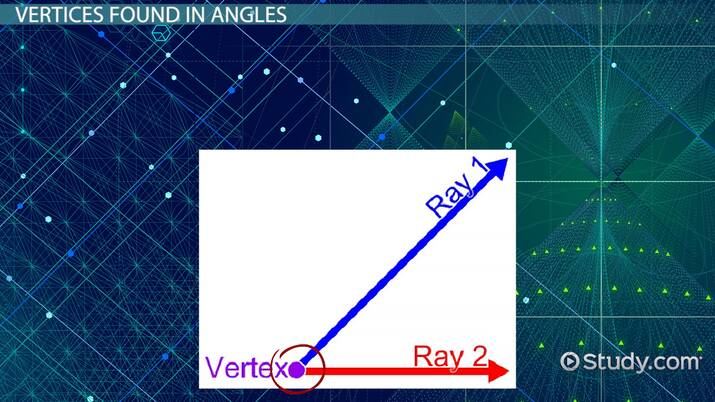Vertices: Lesson for Kids

Lesson Transcript
Instructor: Jennifer Lowery

Jennifer has taught elementary levels K-3 and has master's degrees in elementary education and curriculum/instruction and educational leadership.

Learn about vertices in geometry, the plural of a vertex. Explore the vertex of an angle, vertices in plane figures or two-dimensional shapes, and vertices in solid figures, which are three-dimensional shapes. Updated: 12/21/2021

What Are Vertices?

Look around the room where you're currently sitting. Did you know that you are surrounded by examples of geometry? Find places where two lines or edges come together, like the corner of a desk, the points on a picture frame, the corners on a tissue box. These are examples of vertices.

A vertex, first of all, is the singular form of 'vertices', and it represents the location where two or more lines or edges are connected.An error occurred trying to load this video.

Try refreshing the page, or contact customer support.

Coming up next: What is a Regular Polygon? - Lesson for Kids

You're on a roll. Keep up the good work!

Replay
Your next lesson will play in 10 seconds
• 0:04 What Are Vertices?
• 0:27 Vertices Found in Angles
• 1:07 Plane Figure Vertices
• 1:39 Vertices Found in…
• 2:16 Lesson Summary
Save Save

Want to watch this again later?

Timeline
Autoplay
Autoplay
Speed Speed

Vertices Found in Angles

Angles are created when two lines or rays come together to form a point. The point where the lines or rays connect is called a vertex. The vertex can be small or big, depending on how far apart the lines or rays are.

• Acute angles are angles that measure smaller than 90 degrees. The vertex in an acute angle will be small because the lines are closer together.
• Obtuse angles measure larger than 90 degrees. In these angles, the vertex will be larger since the lines or rays are farther apart.
• A right angle measures exactly 90 degrees; no more and no less. You can fit a perfect square inside of this vertex. It looks a lot like the letter L!

Plane Figure Vertices

Plane figures are flat shapes that have more than one side. Triangles, squares, and trapezoids are a few examples of plane figures, also sometimes called polygons. These figures are created using lines, and there are places where the lines intersect, which simply is another word for connecting.

A common way to describe them would be corners. For example, a square has four vertices, or corners, since there are four places where the sides connect to each other. A triangle has three vertices. The more sides a shape has, the more vertices it also has.

Vertices Found in Solid Figures

A solid figure is a three-dimensional shape, like a cube, or a cone, or a sphere. These shapes are made of flat or curved faces, edges where the faces come together, and vertices.

To unlock this lesson you must be a Study.com Member.

Register to view this lesson

Are you a student or a teacher?